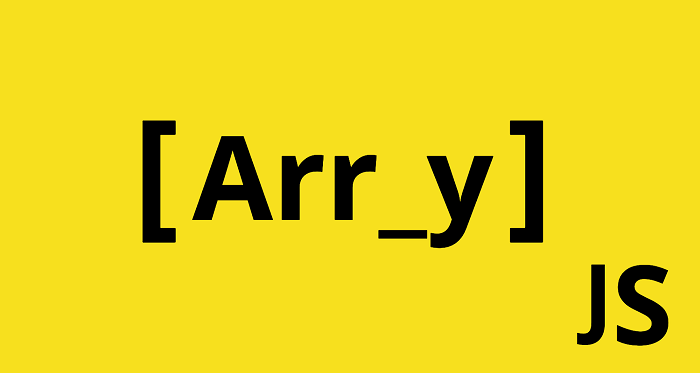# How to Get Unique Values from Array in JavaScript

JavaScript allows you to store data using arrays. It is one of the most commonly used data structures by JS developers. Often you may need to remove duplicate items from JS array. Although JS arrays offer many useful features such as pop(), there is no straight forward function available to filter out duplicates from JS array. In this article, we will learn how to get unique values from Array in JavaScript.

## How to Get Unique Values from Array in JavaScript

Here are the steps to get unique value from array in JavaScript. Let us say you have the following JS array.

`var a = ['a', 1, 'a', 2, '1'];`

First we define a JS function that loops through the array and checks each element to see if it is the first element of the array.

```function onlyUnique(value, index, self) {
return self.indexOf(value) === index;
}
```

The above function checks if a given value is first occurring or not. Then we use the native filter() method available for each array, that loops through the array and leaves behind only those elements that return true when processed by onlyUnique function.

`var unique = a.filter(onlyUnique);`

Here is the result you will get when you run the above code.

`console.log(unique); // ['a', 1, 2, '1']`

Here is putting together the entire code.

```function onlyUnique(value, index, self) {
return self.indexOf(value) === index;
}

// usage example:
var a = ['a', 1, 'a', 2, '1'];
var unique = a.filter(onlyUnique);

console.log(unique); // ['a', 1, 2, '1']```

The above solution works without any third party libraries like JQuery. Also, it works on arrays with mixed data types too.

The above code retains the first occurrence of value. If you want to retain the last occurrence of value, replace indexOf() with lastIndexOf().

On browsers that support ES6, you can shorten the above code to the following.

```// usage example:
var myArray = ['a', 1, 'a', 2, '1'];
var unique = myArray.filter((v, i, a) => a.indexOf(v) === i);

console.log(unique); // unique is ['a', 1, 2, '1']```

In this article, we have learnt how to get unique value from array in JavaScript. You can use it to easily get rid of duplicate values in JS arrays.